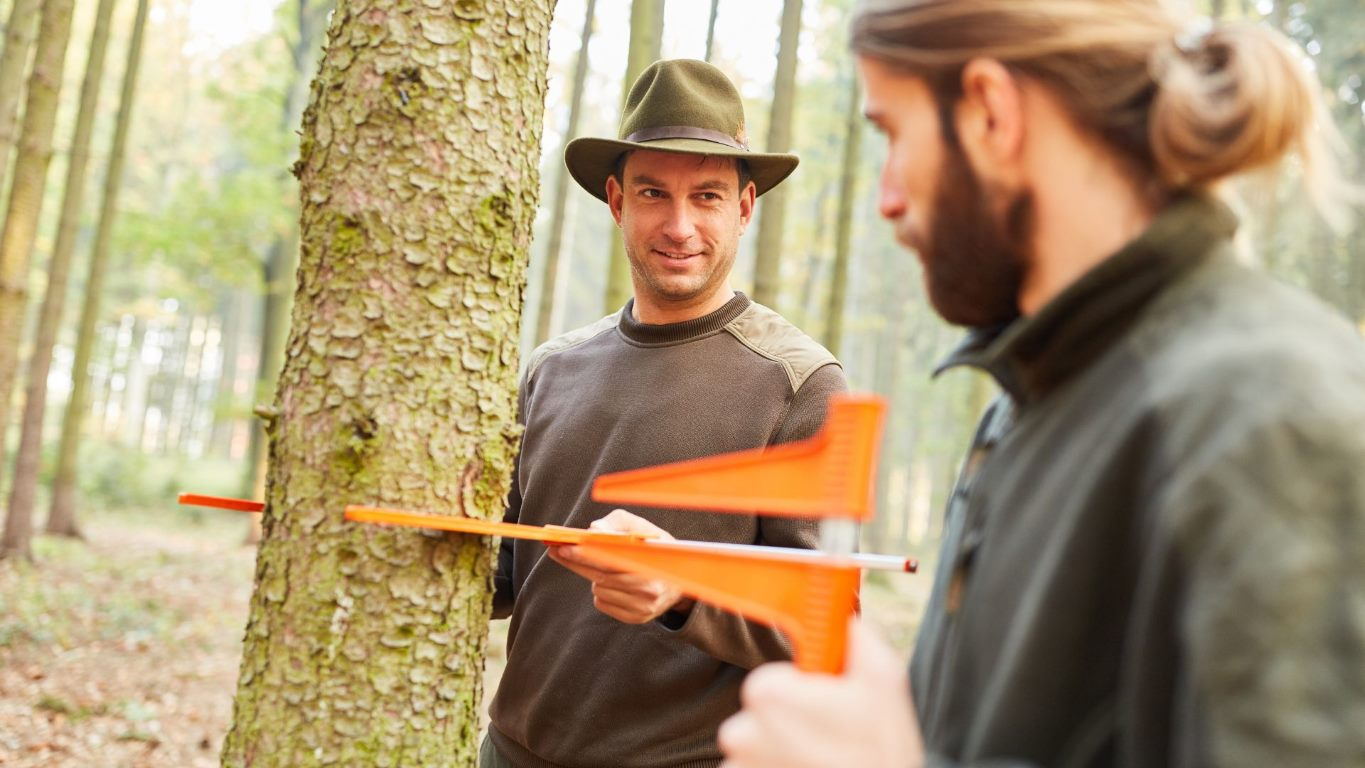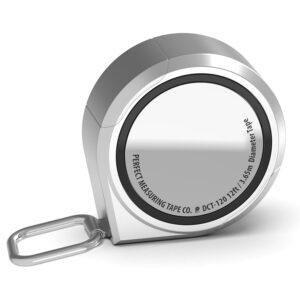As an Amazon Affiliate, We Earn From Qualifying Sales. Read Full Disclosure Here

Have you heard the term DBH thrown around but have no idea what it means? In this article, I will explain what DBH is and how you measure it.

DBH is an abbreviation for Diameter at Breast Height. The diameter at breast height is a standard form of measuring the diameter of a tree. To measure a tree’s DBH, measure the tree’s circumference at breast height. Next, divide the circumference of the tree by Pi.

Being an Arborist, I need to measure DBHs frequently. This article will teach you some trade tricks to make measuring the DBH easier and quicker. Also, I will cover how to measure the DBH on forked and multi-stemmed trees.

Everything Arboriculture is supported by its readers. When you buy through links on this site, we may earn an affiliate commission.

### What Is the DBH of a Tree?

The DBH is a standard unit of measurement in Arboriculture. DBH stands for Diameter at Breast Height.

Trees vary in shape and size, so there was a need for a consistent way to measure the diameter of trees. Additionally, there was a need to measure the diameter of a tree without cutting it down. Thus, the DBH was adopted.

The earliest mention of DBH is in a German text, dated back to 1835.

Measuring a tree at breast height is a convenient and accessible way to measure a tree. It would be too difficult to measure the tree higher up.

The formula for measuring the DBH of a tree is:

DBH = Circumference at Breast Height divided by Pi (3.14)

Though DBH is a standard unit of measurement, the definition of the breast varies by country. So, it’s essential to know what definition of breast your country uses for consistency.

Without a standard height to measure the diameter of a tree, results could vary significantly.

The breast height of a tall person will be different from that of a short person. If these two people measured the same tree using their breast height as a reference, they would get different results.

Here’s a table showing what height each country determines breast to be:

#### So, What Is the DBH Used For?

Measuring a tree’s DBH is the first step in figuring out many different calculations. Some of these calculations include:

• Estimating the volume of a tree
• How much wood a tree will produce
• Biomass
• How much carbon a tree is storing
• How big a tree protection zone needs to be

### How Do You Calculate the DBH of a Tree

The easiest way to measure the DBH of a tree is with a diameter/circumference measuring tape. So, all you need to do is measure out where the breast height is. Then, measure around the tree. One side of the tape will tell you the circumference, and the other side will tell you the diameter.#### Perfect Pi Diameter Circumference Pi Tape Measure

A diameter/circumference tape measure. Includes inches and the metric system, making it easy for anyone to use

Tip: To save time, measure the breast height on yourself for reference. Now by standing next to a tree, you will know where the breast height is without having to measure it every time.

However, some trees will require a little more work.

#### How to Measure the DBH of Different Types of Trunks: Step-by-Step

Here I will teach you how to measure the DBH of different trunks. I will assume you use a standard soft measuring tape for these explanations.

##### Single-Stemmed Tree on Level Ground
1. Use the measuring tape to mark the breast height on the trunk. Remember, this measurement will vary by country.
2. Wrap the tape around the tree to get the circumference. Ensure the tape is straight and tight.
3. To get the DBH, divide the circumference by Pi (3.14)
##### Single-Stemmed Tree on a Slopped Ground
1. Find the center of the trunk at ground level. This point will be between the highest and lowest points of the slope.
2. From this point, measure out the breast height on the trunk.
3. Wrap the tape tightly around the trunk to get the circumference.
4. To get the DBH, divide the circumference by Pi (3.14)
##### Single-Stemmed Tree on an Angle
1. Find the center of the trunk at ground level
2. From this point, measure out the breast height on the trunk. However, follow the angle of the trunk when measuring. Do not measure straight up.
3. From breast height, measure around the tree to get the circumference.
4. Divide the circumference by Pi (3.14) to get the DBH
##### Trees With a Split Trunk

Trees with a split trunk will be measured the same way as a single-stemmed trunk. However, if the splitting of the trunk happens below breast height, you will need to measure before the split.

So, in instances where there’s a split, you might measure the circumference at 2ft rather than 4.5ft.

##### Multi-Stemmed Trees
1. Determine the breast height of each stem
2. Measure the circumference of the first stem, then divide that number by Pi (3.14)
3. Repeat Step 2 until you have the DBH of each stem
4. Cube each DBH (times the DBH by itself)
5. Add all the cubed DBHs together
6. Once you have added all the DBHs, find the square root of that number. Then, round up your result, and you will have the DBH of your tree

For example:

• DBH of the different stems: 4″ 5″ 7″ 4″
• Cube these numbers: 4, 5, 7 and 4: 16, 25, 49 and 16
• Add these numbers together: 16 + 25 + 49 + 16 = 106
• Find the square root of 106 = 10.29
• Round up to get the DBH = 11